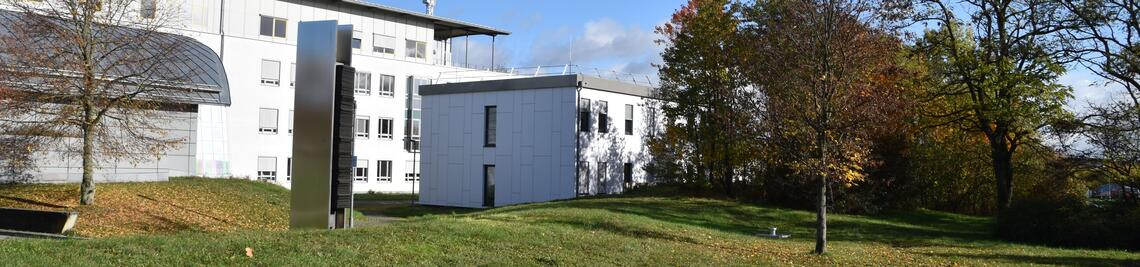•# WCRP 2018

## ICALP Workshop: Constrained Recognition Problems

-- Organizers: Steven Chaplick  and Ignaz Rutter

## Monday 9-July-2018. Prague, Czech Republic.

-- to occur during the 45th International Colloquium on Automata, Languages, and Programming (ICALP)

Information regarding registration can be found on the ICALP webpage here.

## Description

Recognition is fundamental class of algorithmic problems in the study of discrete structures. Classical examples include testing planarity and testing whether a graph is an interval graph. Often, the goal of such a recognition problem is to construct a representation certifying membership in the class, e.g., a planar embedding or intersection representation by intervals of the real number line. For the past 15 years, this fundamental class of problems has attained a renaissance period where several generalizations have gained prominence. Two primary examples are the classes of graph editing problems and partial representation extension problems. The former widens the class of graphs by allowing k modification operations which can be applied to an input graph in order to fit it into the class. The latter constrains the sought representation by prescribing the exact representation for a subgraph. Recently, there has been significant progress on the editing problems for several standard classes of graphs, e.g., interval graphs [SODA 2016], chordal graphs [SODA 2017, Algorithmica 2016], H-minor free graphs [FOCS 2012], and planar graphs [SODA 2017]. The partial representation extension problem has also been studied for various graph classes, among others planar graphs [TALG 2015], level-planar graphs [SODA 2017], interval graphs [TALG 2015, Algorithmica 2017], and bar visibility graphs [Algorithmica 2017]. The purpose of this workshop is to describe the main techniques employed to obtain recent significant results as well as to highlight the key challenges in these areas.

## Program (14:00 - 17:30)

14:00-15:30 Partial Representation Extension
• 14:00-15:00 Jan Kratochvíl -- Department of Applied Mathematics, Charles University (Prague, Czech Republic)
Title: Geometric Representations of Graphs: Representation Extension versus Simultaneous Representations
Abstract: Classes of intersection and contact graphs of geometric objects are well studied both for their relevance to graph visualization and for their interesting structural and algorithmic properties. Many of them can be recognized in polynomial time (e.g., interval graphs, circle graphs, permutation graphs and many more). The aim of the talk is to compare two natural generalizations of the recognition problem: RepExt (when a part of the input graph comes pre-represented) and SimRep (when two or more input graphs are to be represented simultaneously, i.e. their common part has to be represented the same way for each of them). In all cases when computational complexity is currently known, SimRep is at least as difficult as RepExt. Is this a coincidence or a law of nature?
• 15:00-15:30 Bartosz Walczak -- Department of Theoretical Computer Science, Jagiellonian University (Kraków, Poland)

Title: Extending partial representations of trapezoid graphs
Abstract: A trapezoid graph is an intersection graph of trapezoids spanned between two horizontal lines. The partial representation extension problem for trapezoid graphs is a generalization of the recognition problem: given a graph G and an assignment ξ of trapezoids to some vertices of G, can ξ be extended to a trapezoid intersection model of the entire graph G? We show that this can be decided in polynomial time. As an important subroutine, we provide a polynomial-time algorithm to extend partial representations of trapezoid orders, which proceeds by reduction to satisfiability of a carefully designed 2-SAT formula. We examine possibilities of applying an analogous idea to circular-arc graphs, which is the major class of geometric intersection graphs for which the complexity of partial representation extension remains unknown. This is joint work with Tomasz Krawczyk.

15:30-16:00 Coffee Break
16:00-17:30 Graph Editing
• 16:00-17:00 MS Ramanujan -- Department of Computer Science, University of Warwick (Warwick, UK)
Title: Graph Editing to SAT Editing.
Abstract: One of the most frequently studied classes of computational problems is the class of graph editing problems where the objective is to perform a series of graph operations on a given graph to achieve a certain graph property. Common operations include adding or removing a prescribed number of vertices or edges to achieve the specified graph property. The significant amount of interest attracted by graph editing problems arises from the fact that a large number of problems arising in theory and practice can be modelled as graph editing problems. Over the years, there has been a systematic study of various subclasses of graph editing problems from the point of view of approximation algorithms, exponential time algorithms and parameterized algorithms, leading to a rich collection of sophisticated algorithmic techniques with varied applications.
In fact, over the last decade, graph modification problems and related techniques have found extensive use in the design of parameterized algorithms for Satisfiability and Constraint Satisfaction Problems by exploiting the notion of backdoor sets. The aim of this talk is to describe the connection between graph editing and SAT solving, survey recent developments and discuss open problems relating the application of graph editing techniques to solve SAT/CSP modification problems, which in turn will lead to new parameterized algorithms for SAT and CSP.
• 17:00-17:30 Yixin Cao -- Department of Computing, Hong Kong Polytechnic University (Hong Kong, China)
Title: Interval graphs and (normal Helly) circular-arc graphs
Abstract: Interval graphs are arguable the simplest and most useful graph class. Circular-arc graphs, extension of interval graphs, are among the most complicated ones: After more than half century, we still do not know their forbidden induced subgraphs. We focus on the "smallest" subclass of circular-arc graphs that still contains all interval graphs. We survey several combinatorial and algorithmic applications of a novel connection between it and interval graphs.